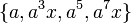# Non-central Z4 in M16

This article is about a particular subgroup in a group, up to equivalence of subgroups (i.e., an isomorphism of groups that induces the corresponding isomorphism of subgroups). The subgroup is (up to isomorphism) cyclic group:Z4 and the group is (up to isomorphism) M16 (see subgroup structure of M16).
The subgroup is a normal subgroup and the quotient group is isomorphic to cyclic group:Z4.
VIEW: Group-subgroup pairs with the same subgroup part | Group-subgroup pairs with the same group part| Group-subgroup pairs with the same quotient part | All pages on particular subgroups in groups

## Definition

We consider the group:$G = M_{16} = \langle a,x \mid a^8 = x^2 = e, xax = a^5 \rangle$

with$e$ denoting the identity element.

This is a group of order 16, with elements:$\! \{ e, a, a^2, a^3, a^4, a^5, a^6, a^7, x, ax, a^2x, a^3x, a^4x, a^5x, a^6x, a^7x \}$

We are interested in the subgroup:$H := \{ e, a^2x, a^4, a^6x \}$

This subgroup is isomorphic to cyclic group:Z4, with generator$a^2x$. It is a normal subgroup and the quotient group is also isomorphic to the cyclic group:Z4.

Here is the multiplication table for$H$. Note that$H$ is an abelian group so we don't have to worry about left/right issues:

Element/element$e$$a^2x$$a^4$$a^6x$$e$$e$$a^2x$$a^4$$a^6x$$a^2x$$a^2x$$a^4$$a^6x$$e$$a^4$$a^4$$a^6$$e$$a^2$$a^6x$$a^6x$$e$$a^2x$$a^4$

## Cosets

The subgroup is a normal subgroup and so its left cosets are the same as its right cosets. It has 4 cosets in the whole group:$\{ e, a^2x, a^4, a^6x \}, \{ a, a^3x, a^5, a^7x \}, \{ a^2, a^4x, a^6, x \}, \{ a^3, a^5x, a^7, ax \}$

Below is the multiplication for$G/H$. It is a cyclic group of order 4 with generator the coset$\{ a, a^3x, a^5, a^7x \}$. Since it is an abelian group, we do not need to worry about left/right convention for the multiplication table:

Element/element$\{ e, a^2x, a^4, a^6x \}$$\{ a, a^3x, a^5, a^7x \}$$\{ a^2, a^4x, a^6, x \}$$\{ a^3, a^5x, a^7, ax \}$$\{ e, a^2x, a^4, a^6x \}$$\{ e, a^2x, a^4, a^6x \}$$\{ a, a^3x, a^5, a^7x \}$$\{ a^2, a^4x, a^6, x \}$$\{ a^3, a^5x, a^7, ax \}$$\{ a, a^3x, a^5, a^7x \}$$\{ a, a^3x, a^5, a^7x \}$$\{ a^2, a^4x, a^6, x \}$$\{ a^3, a^5x, a^7, ax \}$$\{ e, a^2x, a^4, a^6x \}$$\{ a^2, a^4x, a^6, x \}$$\{ a^2, a^4x, a^6, x \}$$\{ a^3, a^5x, a^7, ax \}$$\{ e, a^2x, a^4, a^6x \}$$\{ a, a^3x, a^5, a^7x \}$$\{ a^3, a^5x, a^7, ax \}$$\{ a^3, a^5x, a^7, ax \}$$\{ e, a^2x, a^4, a^6x \}$$\{ a, a^3x, a^5, a^7x \}$$\{ a^2, a^4x, a^6, x \}$

## Subgroup properties

### Invariance under automorphisms and endomorphisms

Property Meaning Satisfied? Explanation
normal subgroup invariant under inner automorphisms Yes Follows from being the only non-central cyclic group of order four.
characteristic subgroup invariant under all automorphisms Yes Follows from being the only non-central cyclic group of order four.
fully invariant subgroup invariant under all endomorphisms No The endomorphism$a \mapsto e, x \mapsto x$ (which is a retraction onto$\langle x \rangle$) does not preserve this subgroup.
isomorph-free subgroup no other isomorphic subgroup No center of M16 is another isomorphic subgroup.
isomorph-characteristic subgroup every isomorphic subgroup is characteristic Yes The only isomorphic subgroups are the subgroup itself and center of M16.

## GAP implementation

The group and subgroup pair can be constructed as follows:

G := SmallGroup(16,6); H := Filtered(NormalSubgroups(G),x -> Order(x) = 4 and IsCyclic(x) and not(x = Center(G)));

Here is the GAP display:

gap> G := SmallGroup(16,6); H := Filtered(NormalSubgroups(G),x -> Order(x) = 4 and IsCyclic(x) and not(x = Center(G)));
<pc group of size 16 with 4 generators>
Group([ f2*f3, f4 ])

Here is GAP code to verify some of the assertions in this page:

gap> Order(G);
16
gap> Order(H);
4
gap> Index(G,H);
4
gap> StructureDescription(H);
"C4"
gap> StructureDescription(G/H);
"C4"
gap> IsNormal(G,H);
true
gap> IsCharacteristicSubgroup(G,H);
true
gap> IsFullinvariant(G,H);
false